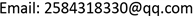CSAComputer Science and Application2161-8801Scientific Research Publishing10.12677/CSA.2020.109167CSA-37661CSA20200900000_32749245.pdf信息通讯 基于贝叶斯网络的无人机任务可靠性建模方法 The Reliability Modeling Method of UAV Mis-sion Based on Bayesian Network 殷鼎212121空军工程大学装备管理与无人机工程学院，陕西 西安null02092020100915881594© Copyright 2014 by authors and Scientific Research Publishing Inc. 2014This work is licensed under the Creative Commons Attribution International License (CC BY). http://creativecommons.org/licenses/by/4.0/

The Reliability Modeling Method of UAV Mission Based on Bayesian Network

Yinding Qian, Ying Wang, Yun Sun

College of Equipment Management and UAV Engineering, Air Force Engineering University, Xi’an ShaanxiReceived: Aug. 29th, 2020; accepted: Sep. 10th, 2020; published: Sep. 17th, 2020ABSTRACT

Due to its inherent dexterity, UAV is not easy to be detected when carrying out activities, and it can often achieve unexpected effects. Even if it is detected and destroyed, the loss will be much less than the loss of human aircraft. Therefore, there is bound to be a place for UAV in future wars. At the same time, the UAV mission reliability is related to the success of the task. In order to explore the reliability of the UAV mission modeling method, this article is based on Bayesian network is studied. First of all, according to the topology of the unmanned aerial vehicle (UAV) each subsystem mission reliability block diagram is established, and on the basis of mission reliability diagram coverted into Bayesian networks. Finally, the feasibility of model is validated by an example. The research in this paper provides support for the follow-up research on the reliability of UAV mission.

Keywords:UAV, Mission Reliability, Bayesian Network

Copyright © 2020 by author(s) and Hans Publishers Inc.

This work is licensed under the Creative Commons Attribution International License (CC BY 4.0).

http://creativecommons.org/licenses/by/4.0/1. 引言

2. 无人机系统

Influence parameters of abnormal and extreme environmental factor

ka电磁环境
kb障碍物
km自然环境

3. 无人机任务可靠性分析

3.1. 控制分系统

P t i = 1 − e − λ i t (1)

P t i = k a ⋅ k b ⋅ k m ( 1 − e − λ i t ) (2)

3.2. 信息传输分系统

P r i = k a ⋅ k b ⋅ k m ⋅ M T B F M T B F + M T T R (3)

3.3. 地面测控分系统

3.4. 任务载荷分系统

3.5. 无人机任务可靠性框图

P i = ∏ i = 1 4 P t i ⋅ ( 1 − ( 1 − P t 4 ) ( 1 − P t 5 ) ) ⋅ ∏ i = 1 3 P r i ⋅ ( 1 − ∏ i = 1 3 ( 1 − P k i ) ) ⋅ P n (4)

4. 贝叶斯网络模型4.1. 贝叶斯网络基本理论

4.2. 无人机任务可靠性的贝叶斯网络建模过程4.2.1. 贝叶斯网络的建立

4.2.2. 贝叶斯网络推理

P ( D ) = ∏ i = 1 4 p ( C i ) (5)

C1和C2分别由B1、B2、B3和B4、B5、B6串联组成，C3由B7、B8、B9并联组成可得：

p ( C 1 ) = p ( B 1 ) ⋅ p ( B 2 ) ⋅ p ( B 3 ) (6)

p ( C 2 ) = p ( B 4 ) ⋅ p ( B 5 ) ⋅ p ( B 6 ) (7)

p ( C 3 ) = 1 − ( 1 − p ( B 7 ) ) ⋅ ( 1 − p ( B 8 ) ) ⋅ ( 1 − p ( B 9 ) ) (8)

p ( B 2 ) = p ( A 1 ) ⋅ p ( A 2 ) ⋅ p ( A 3 ) (9)

p ( B 3 ) = 1 − ( 1 − p ( A 4 ) ) ⋅ ( 1 − p ( A 6 ) ) (10)

5. 建模与计算

Failure probability of the origina

6. 结束语